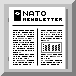........ published in NEWSLETTER # 69

ALGEBRAIC GROUPS AND THEIR REPRESENTATIONS
By Professor R. W. Carter, University of Warwick, Coventry (U.K.)

This volume (NATO ASI SERIES C517) contains 19 articles on the structure and representation theory of algebraic groups by a team of distinguished authors. The content can be divided into representation theory, subgroup structure, and classification problems.

An article by Steinberg gives a new and much shortened proof of the isomorphism and isogeny theorems for connected reductive groups. To any such group over an algebraically closed field there is a combinatorial object called its root datum. Every isomorphism of connected reductive groups induces an isomorphism of root data and the isomorphism theorem asserts that, conversely every isomorphism of root data arises from an isomorphism of connected reductive groups.

Lusztig describes a new approach to the study of unipotent characters of finite reductive groups which, it is hoped, will give a general explanation of why the theory of unipotent characters depends only on the Weyl group with Frobenius action, and not on the root datum or the number of elements in the finite field. Carter contributes a survey article on results on representations of simple Lie algebras which come from the theory of quantum groups. Such results include those arising from Littelmann's path model. An article by Littelmann describes the path model, which gives an approach to standard monomial theory entirely free from case by case considerations, while Tanisaki obtains new results identifying representations arising from parabolic subgroups with abelian unipotent radical.

A main aim of the modular representation theory of algebraic groups is to prove Lusztig's conjectured character formula, which is the analogue of Weyl's character formula in characteristic p. It is known to be true when p is sufficiently large, but it is not known how large p has to be. An article by Scott gives the background to Lusztig's character formula, and and Anderson describes a possible approach to ist proof using tilting modules.

There are two articles on the modular representation theory of the symmetric groups by James and Kleshchev. The characters of the irreducible modules are unknown in this situation also. James contributes a survey article on Specht modules, the Murphy basis, and Schur algebras. Kleshchev describes recent results on branching numbers obtained by restriction from Sn to Sn-1, with application to his proof of the Mullineux conjecture concerning tensoring with the sign representation. An article by Cline, Parshall and Scott on endomorphism algebras concentrates on the theory of stratified algebras with applications to the cross characteristic representation theory of finite reductive groups.

An article by McNinch discusses the extent to which semisimplicity survives for representations in characteristic p.

Robinson contributes a survey article on the block theory of modular representations of finite groups, including Alperin's conjecture on weights and Broue's conjecture on blocks with abelian defect group. The latter is closely linked to the Deligne - Lusztig character theory of finite reductive groups.

The volume also contains several articles on the subgroup structure of simple algebraic groups and the associated finite simple groups of Lie type. Articles by Seitz and Brundan are concerned with double cosets in reductive groups. Seitz discusses the problem of determining pairs of closed subgroups with only finitely many double cosets. Brundan discusses the related problem of finding pairs of closed subgroups with a dense double coset.

An article by Liebeck discusses subgroups of the exceptional simple algebraic groups and the finite exceptional groups. Some very remarkable embeddings of finite groups in algebraic groups are seen to arise. There is an article by Saxl describing all subgroups containing a given element of a specific type, such as a regular unipotent element or a bireflection. Then an article by Guralnick discusses various applications of the subgroup structure of algebraic groups. Such applications include probabilistic questions on the generation of simple groups, and also geometric questions related to coverings of curves.

There is then an article by Aschbacher on the classification of quasithin finite simple groups. The classification of finite simple groups is of critical importance in group theory. A major revision of the proof of the classification theorm is under way. The treatment of quasithin groups was one of the last steps in the proof. A lengthy but incomplete manuscript on this part was written by Mason. A new version is needed to fill the gaps and Aschbacher, Smith and Meierfrankenfeld are well advanced in producing this. Aschbacher's article outlines this work.

The volume concludes with an article by Borovik describing joint work with Altinel, Cherlin and Corredor on the classification of simple groups with finite groups with finite Morley rank. The main conjecture is that simple infinite groups of finite Morley rank should be simple algebraic groups over algebraically closed fields.

This volume, including as it does articles by the leading workers in the subject, contains a wealth of recent ideas and results on algebraic groups and related finite groups.
Reference books: B43, C429, C466, C471, C517

